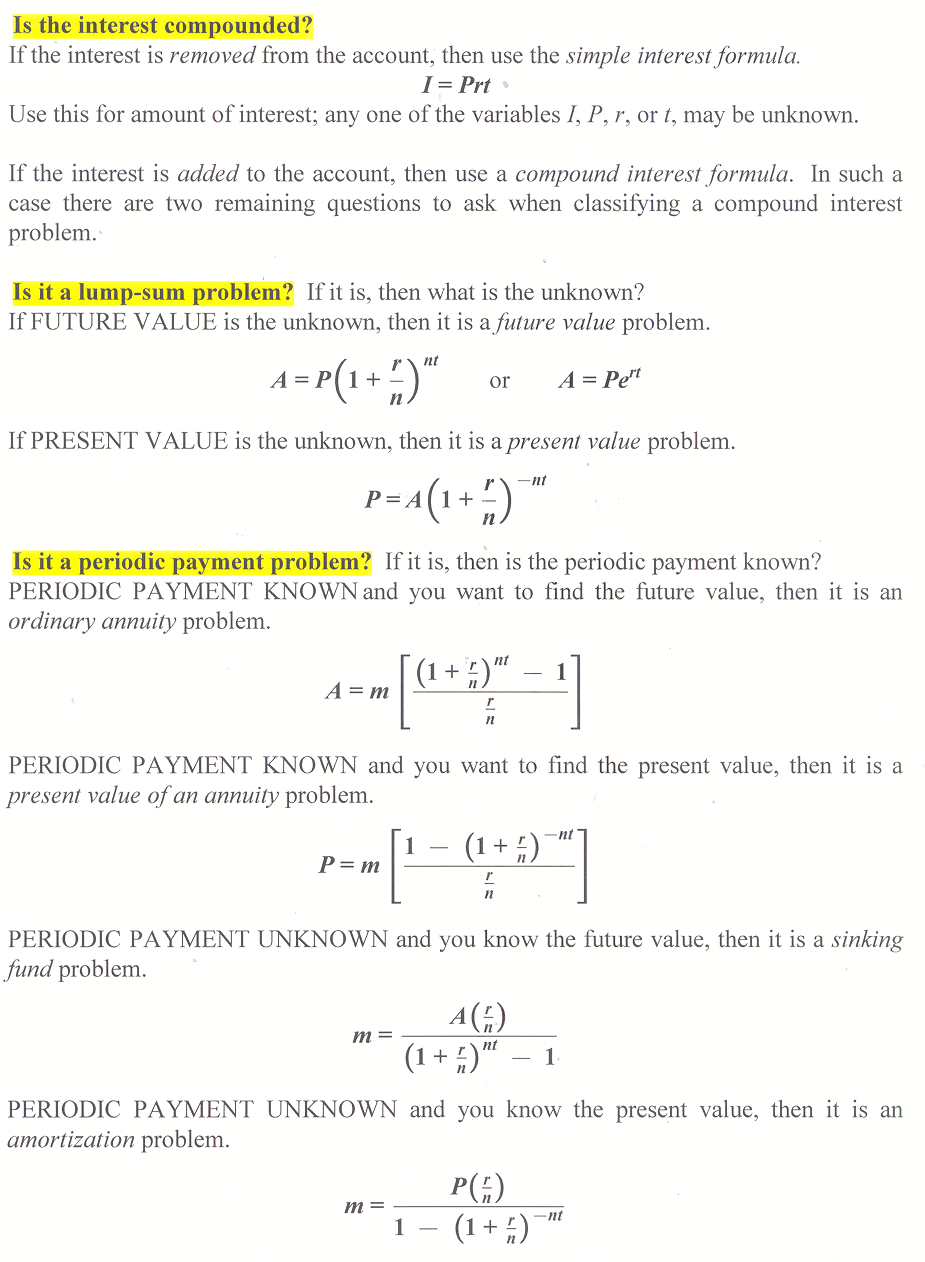## 11.7 Outline

1. Definition of variables
2. Classification of financial formulas
1. simple interest formula
2. compound interest formula
3. future value problem
4. present value problem
5. ordinary annuity
6. present value of an annuity
7. sinking fund
8. amortization

## 11.7 Essential IdeasSee the whole entry

## 5.1 Outline

1. Basic concepts
1. counting numbers
2. natural numbers